Next: Fourier series of the Up: Classical waveforms Previous: Sawtooth waves and symmetry   Contents   Index

# Dissecting classical waveforms

Among the several conclusions we can draw from the even/odd harmonic decomposition of the sawtooth wave (Figure 10.2), one is that a square wave can be decomposed into a linear combination of two sawtooth waves. We can carry this idea further, and show how to compose any classical waveform having only jumps (discontinuities in value) but no corners (discontinuities in slope) as a sum of sawtooth waves of various phases and amplitudes. We then develop the idea further, showing how to generate waveforms with corners (either in addition to, or instead of, jumps) using another elementary waveform we'll call the parabolic wave.

Suppose first that a waveform of periodhas discontinuities at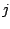different points,, all lying on the cycle between 0 and, at which the waveform jumps by values. A negative value of, for instance, would mean that the waveform jumps from a higher to a lower value at the point, and a positive value ofwould mean a jump from a lower to a higher value.For instance, Figure 10.3 (part a) shows a classical waveform with two jumps: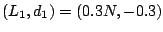and. Parts (b) and (c) show sawtooth waves, each with one of the two jumps. The sum of the two sawtooth waves reconstructs the waveform of part (a), except for a possible constant (DC) offset.

The sawtooth wave with a jump of one unit at the point zero is given by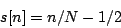over the period, and repeats for other values of. A sawtooth wave with a jumpis given by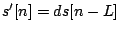. The sum of all the component sawtooth waves is:The slopes of the segments of the waveform of part (a) of the figure are all the same, equal to the sum of the slopes of the component sawtooth waves:Square and rectangle waves have horizontal line segments (slope zero); for this to happen in general the jumps must add to zero:.To decompose classical waveforms with corners we use the parabolic wave, which, over a single period from 0 to, is equal to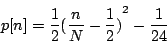as shown in Figure 10.4. It is a second-degree (quadratic) polynomial in the variable, arranged so that it reaches a maximum halfway through the cycle at, the DC component is zero (or in other words, the average value over one cycle of the waveform is zero), and so that the slope changes discontinuously by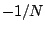at the beginning of the cycle.

To construct a waveform with any desired number of corners (suppose they are at the points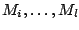, with slope changes equal to), we sum up the necessary parabolic waves: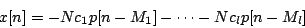An example is shown graphically in Figure 10.5.If the sum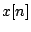is to contain line segments (not segments of curves), theterms in the sum must sum to zero. From the expansion ofabove, this implies that. Sums obtained from existing classical waveforms (as in the figure) will always satisfy this condition because the changes in slope, over a cycle, must all add to zero for the waveform to connect with itself.Next: Fourier series of the Up: Classical waveforms Previous: Sawtooth waves and symmetry   Contents   Index
Miller Puckette 2006-12-30